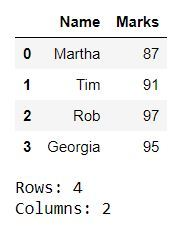# Count the number of rows and columns of Pandas dataframe

In this article, we’ll see how we can get the count of the total number of rows and columns in a Pandas DataFrame. There are different methods by which we can do this. Let’s see all these methods with the help of examples.

Example 1: We can use the `dataframe.shape` to get the count of rows and columns. `dataframe.shape` and `dataframe.shape` gives count of rows and columns respectively.

 `# importing the module ` `import` `pandas as pd ` ` `  `# creating a DataFrame ` `dict` `=` `{``'Name'` `: [``'Martha'``, ``'Tim'``, ``'Rob'``, ``'Georgia'``], ` `        ``'Marks'` `: [``87``, ``91``, ``97``, ``95``]} ` `df ``=` `pd.DataFrame(``dict``) ` ` `  `# displaying the DataFrame ` `display(df) ` ` `  `# fetching the number of rows and columns ` `rows ``=` `df.shape[``0``] ` `cols ``=` `df.shape[``1``] ` ` `  `# displaying the number of rows and columns ` `print``(``"Rows: "` `+` `str``(rows)) ` `print``(``"Columns: "` `+` `str``(cols)) `

Output :Example 2 : We can use the `len()` method to get the count of rows and columns. `dataframe.axes` represents rows and `dataframe.axes` represents columns. So, `dataframe.axes` and `dataframe.axes` gives the count of rows and columns respectively.

 `# importing the module ` `import` `pandas as pd ` ` `  `# creating a DataFrame ` `dict` `=` `{``'Name'``:[``'Martha'``, ``'Tim'``, ``'Rob'``, ``'Georgia'``], ` `        ``'Marks'``:[``87``, ``91``, ``97``, ``95``]} ` `df ``=` `pd.DataFrame(``dict``) ` ` `  `# displaying the DataFrame ` `display(df) ` ` `  `# fetching the number of rows and columns ` `rows ``=` `len``(df.axes[``0``]) ` `cols ``=` `len``(df.axes[``1``]) ` ` `  `# displaying the number of rows and columns ` `print``(``"Rows: "` `+` `str``(rows)) ` `print``(``"Columns: "` `+` `str``(cols)) `

Output :Example 3 : Similar to the example 2, `dataframe.index `represents rows and` dataframe.columns `represents columns. So, `len(dataframe.index)` and `len(dataframe.columns)` gives count of rows and columns respectively.

 `# importing the module ` `import` `pandas as pd ` ` `  `# creating a DataFrame ` `dict` `=` `{``'Name'``:[``'Martha'``, ``'Tim'``, ``'Rob'``, ``'Georgia'``], ` `        ``'Marks'``:[``87``, ``91``, ``97``, ``95``]} ` `df ``=` `pd.DataFrame(``dict``) ` ` `  `# displaying the DataFrame ` `display(df) ` ` `  `# fetching the number of rows and columns ` `rows ``=` `len``(df.index) ` `cols ``=` `len``(df.columns) ` ` `  `# displaying the number of rows and columns ` `print``(``"Rows: "` `+` `str``(rows)) ` `print``(``"Columns: "` `+` `str``(cols)) `

Output :My Personal Notes arrow_drop_upCheck out this Author's contributed articles.

If you like GeeksforGeeks and would like to contribute, you can also write an article using contribute.geeksforgeeks.org or mail your article to contribute@geeksforgeeks.org. See your article appearing on the GeeksforGeeks main page and help other Geeks.

Please Improve this article if you find anything incorrect by clicking on the "Improve Article" button below.

Article Tags :

Be the First to upvote.

Please write to us at contribute@geeksforgeeks.org to report any issue with the above content.